3.4Simulation Results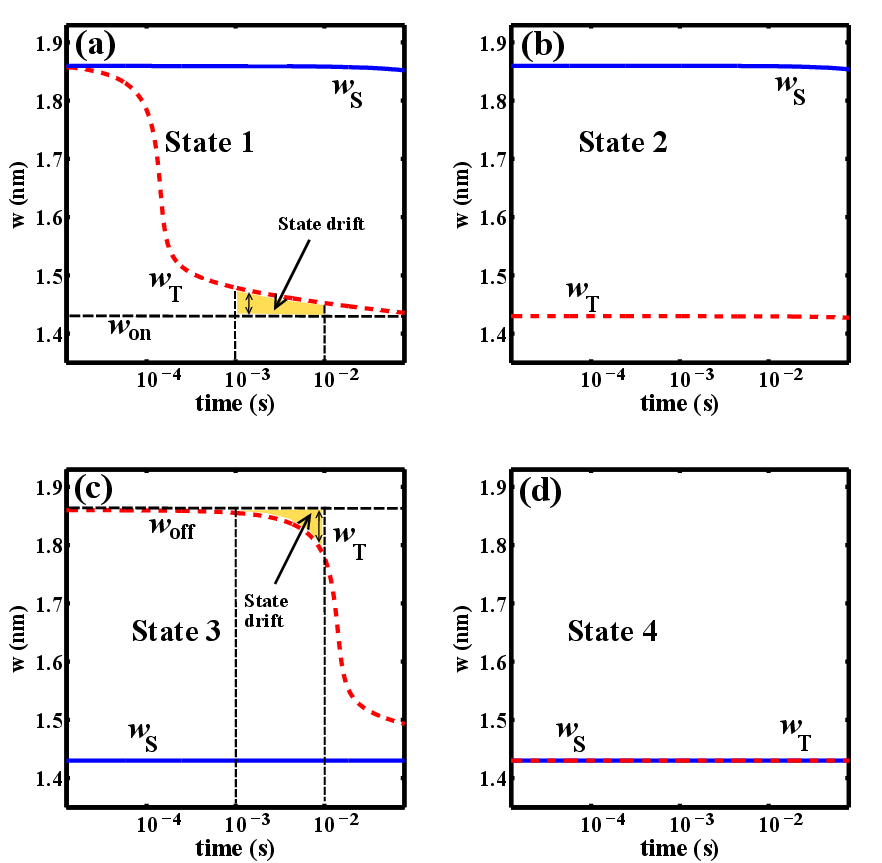Figure 3.6.: Modulation of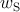and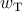during the logic operation for different input patterns.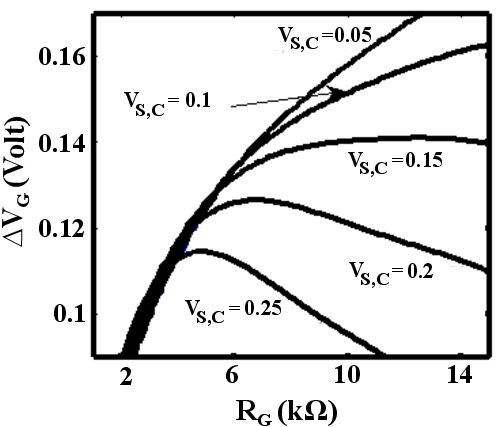Figure 3.7.: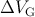as a function of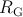for different values of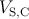.

In order to analyze the TiO-based memristive circuit (Fig. 3.2), the nonlinear model is used for each TiOmemristive switch and thus, coupled with the equation Eq. 3.4, Eq. 3.13–Eq. 3.22 are numerically solved for both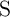and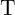. Fig. 3.6 shows the modulation of the tunnel barrier widths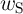and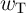during the implication operation for all possible input patterns (State 1–State 4) described in Table 3.2. It illustrates that for pulse durations between 1–10 ms, only in State 1, the target memristor (T) is switched and in all other cases bothand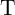are left unchanged. Accordingly, correct logic behavior is achieved for all input states and the logic result is stored as the final resistance state of. Here, the initial tunnel barriers are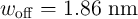and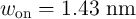which are equivalent to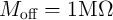and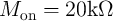at the readout voltage of 0.2 V (Fig. 3.4). The circuit parameters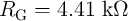,, and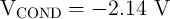are optimized to minimize the SDE as is explained below.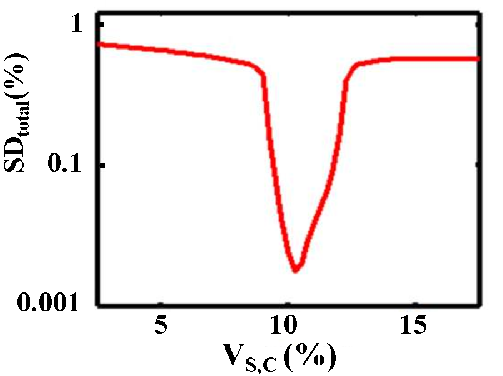Figure 3.8.: Total state drift as a function of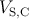.

According to Fig. 3.6, the dominant SDs occur in State 1 (Fig. 3.6a) in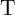(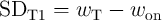) and in State 3 (Fig. 3.6c) in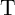(). Therefore, maximizing the modulation of the voltage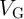between State 3 and State 1 (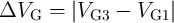) minimizes the possible SDEs in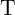. Fig. 3.7 shows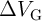as a function of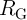for different values of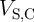where(3.23)

As follows from Fig. 3.7, the optimum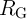corresponds to the maximum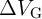which maximizes the modulation of the voltage drop on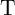between State 3 and State 1 and thus minimizes the SDEs in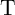shown in Fig. 3.6. Therefore, it is uniquely defined by the memristor’s properties,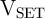and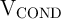. By using Fig. 3.7, an optimum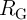is obtained for each value of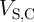and then one can optimize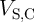to minimize the gate error (Fig. 3.8).

In fact, the voltage modulation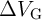increases with increased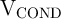and minimizes the SD in memristor. However, an increase in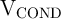results in an increasing error on memristor S, because it tends to switch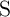in State 1 (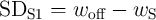) and State 2 (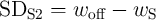). Therefore, there is an optimum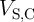for which the total state drift () defined as normalized root mean square error as shown in Fig. 3.8. Optimum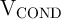and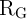are determined at any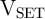by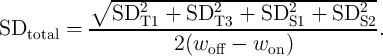(3.24)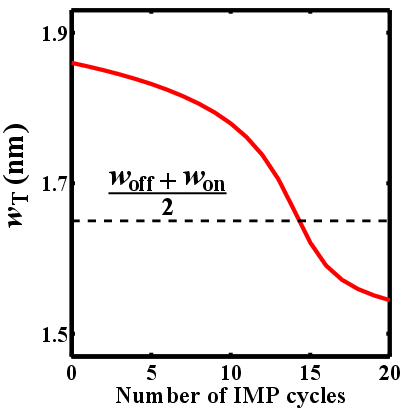Figure 3.9.: Cumulative state drift effect in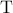for State 3.

TiOmemristive switches enable stateful implication logic by serving simultaneously as non-volatile memory and logic gates. Although the digital data is stored in the high- and low-resistance state of the memristive device, the internal state variableshows analog behavior (Fig. 3.6). Therefore, during the logic operations the voltage drops on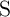and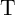tend to push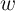toward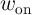, also when their switching is undesired. This causes the state drift error, which accumulates in sequential logic steps and results in a one-bit error after a certain number of implication operations. Thus, refreshing circuitry is required to avoid this error . Fig. 3.9 shows the cumulative SD in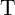during 20 implication operations with 1 ms pulse duration when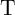and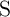are in high and low resistance states, respectively (State 3). It illustrates that after 14 steps the sate variable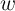is equal to the median value of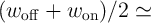1.65 nm which can be readout either as high- or low-resistance state. Whereas any resistance switching in State 3 is considered as an undesired switching, the initial logic state of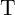has to be rewritten beforereaches 1.65 nm. It is worth mentioning that the linear model predicts a SD of 48.9%  for a particular design example which means a refreshing is required after each implication operation. Compared to the nonlinear ionic drift model, the linear drift model exhibits higher state drift values since it assumes that the state drift is directly proportional to the current or voltage of the memristive devices. However, according to experimental data, the ionic drift velocity shows an exponential dependence on the applied current or voltage  which is taken into account in the nonlinear model by Eq. 3.20 and Eq. 3.21. Once again one has to note that, as high switching voltages are used for (high-speed) computing, the memristor nonlinear model has to be used to take the tunneling effect and dynamical memristor behavior into account.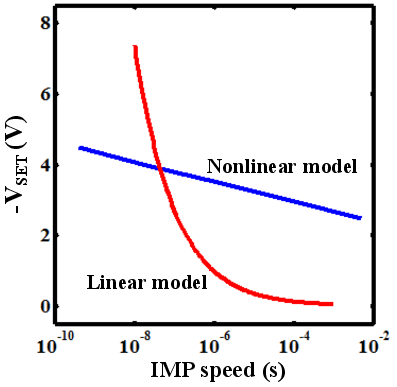Figure 3.10.: Optimized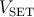pulse amplitude as a function of the pulse duration (IMP speed) based on the linear and the nonlinear memristor models.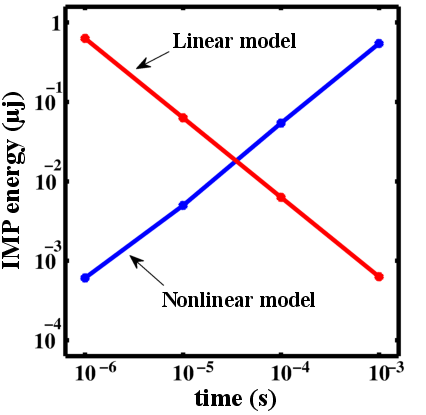Figure 3.11.: Average implication operation energy () as a function of the IMP speed based on the linear and the nonlinear memristor models.

Fig. 3.10 shows only a slight increase of the optimized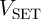pulse amplitude with the implication switching time decreased in contrast to the linear model. This results in large power consumption benefits at higher IMP speed (Fig. 3.11) and shows a good agreement between simulations based on the nonlinear model and the available experimental data (see Fig. S5(b) in Supplementary Information of ) which demonstrates a decrease in switching energy of a TiOmemristive device with the pulse duration decreased. In fact, Fig. 3.11 demonstrates that when the linear model is used, event the trend of the average implication energy consumption (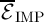) is wrongly predicted as it shows an increase with the IMP speed increased.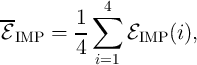(3.25)

where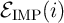denotes the implication energy consumption when the memristive devices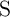and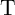are initially in State.